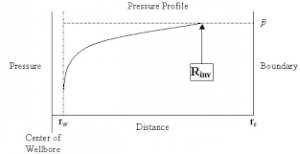## How to Calculate and Solve for Reservoir Radius and Well Spacing | The Calculator EncyclopediaThe image above represents the reservoir radius.

To compute for the reservoir radius, one essential parameter is needed and this parameter is Well Spacing (A).

The formula for calculating the reservoir radius:

re = √[43560A  / π]

Where;

A = Well Spacing

Let’s solve an example;
Given that the well spacing of an reservoir radius is 42. Find the reservoir radius?

This implies that;

A = Well Spacing = 42

re = √[43560A  / π]
re = √[43560 x 42 / π]
re = √[1829520 / π]
re = √582354.3
re = 763.12

Therefore, the reservoir radius is 763.12 ft.